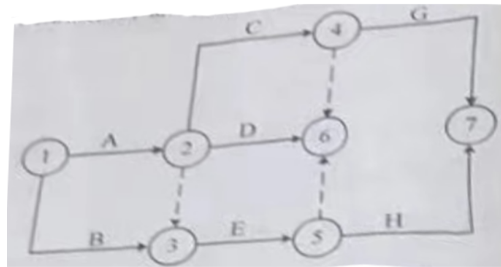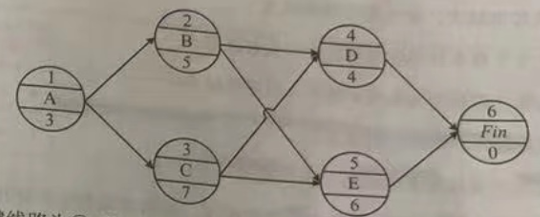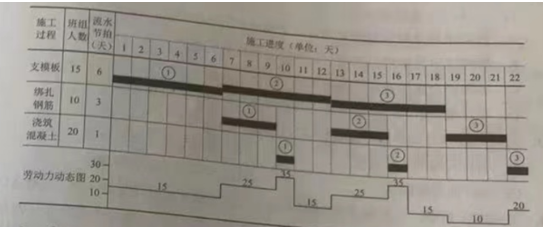163:002022年补考《建设工程项目管理》真题

1
(单项选择题)

• A.

业主方的项目管理才能称为建设工程管理

• B.

建设工程管理的工作仅限于项目实施期的工作

• C.

建设工程管理的核心任务是项目的目标控制

• D.

建设工程管理涉及参与工程项目的各方对工程的管理

• A
• B
• C
• D

2
(单项选择题)

• A.

业主方的项目管理工作涉及项目实施阶段的全过程

• B.

建设工程项目管理的核心任务是为项目的建设和使用增值

• C.

注册建造师的业务范围限于项目实施阶段的项目管理工作

• D.

只有施工企业对项目实施的管理才能称为施工方的项目管理

• A
• B
• C
• D

3
(单项选择题)

• A.

项目结构图

• B.

合同结构图

• C.

工作流程图

• D.

工作任务分工表

• A
• B
• C
• D

4
(单项选择题)

• A.

项目管理工作流程

• B.

项目风险管理与工程保险方案

• C.

项目实施期管理总体方案

• D.

技术方案分析和论证

• A
• B
• C
• D

5
(单项选择题)

• A.

定义项目开发或建设的任务和意义

• B.

确定如何组织该项目的开发或建设

• C.

确定项目建设的目的和指导思想

• D.

确定建设项目的建设目标

• A
• B
• C
• D

6
(单项选择题)

• A.

启动

• B.

设计

• C.

策划

• D.

施工

• A
• B
• C
• D

7
(单项选择题)

• A.

①②③④⑤

• B.

①③②⑤④

• C.

③②①⑤④

• D.

③①②⑤④

• A
• B
• C
• D

8
(单项选择题)

• A.

项目负责人

• B.

施工单位技术负责人

• C.

施工单位负责人

• D.

项目技术负责人

• A
• B
• C
• D

9
(单项选择题)

• A.

参与组建项目管理机构

• B.

对各类资源进行质量监控和动态管理

• C.

主持项目管理机构工作

• D.

决定授权范围内的项目资源使用

• A
• B
• C
• D

10
(单项选择题)

• A.

30

• B.

15

• C.

45

• D.

60

• A
• B
• C
• D

11
(单项选择题)

• A.

站在绝对公平的立场协调业主和承包商的利益

• B.

在维护业主利益的同时不损害承包商的利益

• C.

在维护承包商利益的同时兼顾业主的利益

• D.

尽最大可能同时维护业主和承包商的利益

• A
• B
• C
• D

12
(单项选择题)

• A.

成本分析

• B.

成本核算

• C.

成本控制

• D.

成本考核

• A
• B
• C
• D

13
(单项选择题)

• A.

竞争性成本计划

• B.

指导性成本计划

• C.

改进性成本计划

• D.

实施性成本计划

• A
• B
• C
• D

14
(单项选择题)

• A.

准确的工程量

• B.

预算定额

• C.

施工组织设计

• D.

发包人认可的变更洽商单

• A
• B
• C
• D

15
(单项选择题)

• A.

6000

• B.

－6000

• C.

14000

• D.

－14000

• A
• B
• C
• D

16
(单项选择题)

• A.

操作简单、收支按需核实

• B.

一般用于项目施工各岗位成本的责任核算

• C.

无需核算工程项目在施工过程中出现的债权债务

• D.

科学严密、覆盖面大

• A
• B
• C
• D

17
(单项选择题)

• A.

先价值量，后实物量；先绝对值，后相对值

• B.

先实物量，后价值量：先相对值，后绝对值

• C.

先价值量，后实物量；先相对值，后绝对值

• D.

先实物量，后价值量：先绝对值，后相对值

• A
• B
• C
• D

18
(单项选择题)

• A.

增加收入，弥补亏损

• B.

采取增收节支措施，防止今后再超支

• C.

从控制支出着手，把超支额压缩到最低限度

• D.

停止生产，等待政策调整

• A
• B
• C
• D

19
(单项选择题)

• A.

编制各层进度计划

• B.

项目结构分析

• C.

编制总进度计划

• D.

报告项目决策者论证结果

• A
• B
• C
• D

20
(单项选择题)

• A.

任何情况下，只能有一个起点节点和一个终点节点

• B.

箭线可以从其他箭线上引出或引入

• C.

节点间的连线必须是实箭线

• D.

任何情况下，不允许出现循环回路

• A
• B
• C
• D

21
(单项选择题)

• A.

关键工作的总时差一定是0

• B.

最长线路上工作的总时差最小

• C.

以关键节点为完成节点的工作，其总时差大于自由时差

• D.

实际持续时间和计划持续时间相同的工作，总时差为0

• A
• B
• C
• D

22
(单项选择题)

• A.

不能重新提出认证申请

• B.

在两年后可以重新提出认证申请

• C.

可以提出申诉，并可在半年后重新提出认证申请

• D.

可以提出申诉，并可在一年后重新提出认证申请

• A
• B
• C
• D

23
(单项选择题)

• A.

特种作业

• B.

专门工艺

• C.

隐蔽工程

• D.

重要部位

• A
• B
• C
• D

24
(单项选择题)

• A.

项目经理

• B.

项目总工

• C.

专业监理工程师

• D.

总监理工程师

• A
• B
• C
• D

25
(单项选择题)

• A.

破坏性试验

• B.

理化试验

• C.

见证检验

• D.

抽样检验

• A
• B
• C
• D

26
(单项选择题)

• A.

特别重大事故

• B.

重大事故

• C.

较大事故

• D.

一般事故

• A
• B
• C
• D

27
(单项选择题)

• A.

管理原因

• B.

人为原因

• C.

技术原因

• D.

经济原因

• A
• B
• C
• D

28
(单项选择题)

• A.

分层数据的统计和分析

• B.

分层的类别和层数

• C.

调查分析的类别和层次划分

• D.

逐层深入的排查和分析

• A
• B
• C
• D

29
(单项选择题)

• A.

国务院发展计划部门

• B.

国务院经济贸易主管部门

• C.

国家发改委

• D.

国务院水利部门

• A
• B
• C
• D

30
(单项选择题)

• A.

施工单位技术负责人和总监理工程师

• B.

施工项目经理和建设单位现场代表

• C.

施工项目经理和专业监理工程师

• D.

施工单位技术负责人和监理单位负责人

• A
• B
• C
• D

31
(单项选择题)

• A.

综合治理

• B.

动态治理

• C.

重点治理

• D.

冗余安全度治理

• A
• B
• C
• D

32
(单项选择题)

• A.

宿舍室内净空高度不得低于2.2m

• B.

宿舍内床铺不得超过2层

• C.

每间宿舍同住人数不得超过20人

• D.

人数较多时可采用通铺

• A
• B
• C
• D

33
(单项选择题)

• A.

应急救灾项目应采用公开招标

• B.

建设工程发包人可以不通过招标选择承包人

• C.

关系社会公共利益的大型基础设施应采用国际竞争性招标

• D.

使用外国政府贷款的工程应采用国内邀请招标

• A
• B
• C
• D

34
(单项选择题)

• A.

2021年9月1日

• B.

2021年6月1日

• C.

2021年12月1日

• D.

2022年6月1日

• A
• B
• C
• D

35
(单项选择题)

• A.

负责施工现场的管理工作，与同一施工现场的其他分包人做好配合协调工作

• B.

已竣工分包工程未交付，分包人应负责已完分包工程的成品保护工作

• C.

就分包范围内的工作，分包人根据需要可与发包人直接联系

• D.

分包人征得发包人的同意，可以将其承包的工程转包给他人

• A
• B
• C
• D

36
(单项选择题)

• A.

为从事危险作业的职工办理意外伤害保险

• B.

负责与监理、设计及有关部门联系，协调现场施工关系

• C.

负责编制专项施工组织设计

• D.

完成施工之前生产、生活临时设施的搭设

• A
• B
• C
• D

37
(单项选择题)

• A.

固定单价合同

• B.

固定总价合同

• C.

可调总价合同

• D.

变动单价合同

• A
• B
• C
• D

38
(单项选择题)

• A.

35

• B.

80

• C.

105

• D.

70

• A
• B
• C
• D

39
(单项选择题)

• A.

支付担保

• B.

预付款担保

• C.

付款担保

• D.

履约担保

• A
• B
• C
• D

40
(单项选择题)

• A.

优化项目管理任务分工

• B.

调整进度控制的方法和手段

• C.

调整项目管理工作流程组织

• D.

落实加快施工进度所需的资金

• A
• B
• C
• D

41
(单项选择题)

• A.

监理人

• B.

设计人

• C.

调解委员会

• D.

发包人

• A
• B
• C
• D

42
(单项选择题)

• A.

总费用法

• B.

修正的总费用法

• C.

实际费用法

• D.

修正的实际费用法

• A
• B
• C
• D

43
(单项选择题)

• A.

相比诉讼方式，仲裁的保密性更高

• B.

调解是应该首选的最常见、最有效的方式

• C.

仲裁实行一裁终局制

• D.

仲裁地点必须在工程所在国

• A
• B
• C
• D

44
(单项选择题)

• A.

项目实施的工作过程

• B.

项目管理工作的任务

• C.

项目信息的内容属性

• D.

项目的分解结构

• A
• B
• C
• D

45
(单项选择题)

• A.

项目信息门户的运行期是项目的实施期

• B.

项目信息门户是各参与方信息交流、共同工作、共同使用和互动的管理工具

• C.

项目信息门户主要用于项目的目标控制

• D.

国际上项目信息门户应用的主流是PSWS模式

• A
• B
• C
• D

46
(单项选择题)

• A.

工期不包括按照合同约定所做的期限变更

• B.

保修期自工程实际竣工验收日期起计算

• C.

招标发包工程以投标截止日前14天的日期为基准日期

• D.

缺陷责任期自工程实际竣工日期起计算

• A
• B
• C
• D

47
(单项选择题)

• A.

及时落实工程变更签证并结算工程款

• B.

编制成本控制工作计划

• C.

确定最合适的施工机械、设备使用方案

• D.

确定合理详细的工作流程

• A
• B
• C
• D

48
(单项选择题)

• A.

1天

• B.

2天

• C.

7天

• D.

6天

• A
• B
• C
• D

49
(单项选择题)

• A.

社会环境因素

• B.

自然环境因素

• C.

管理环境因素

• D.

作业环境因素

• A
• B
• C
• D

50
(单项选择题)

• A.

约束机制

• B.

反馈机制

• C.

动力机制

• D.

持续改进机制

• A
• B
• C
• D

51
(单项选择题)

• A.

监理单位

• B.

建设单位

• C.

审图单位

• D.

施工单位

• A
• B
• C
• D

52
(单项选择题)

• A.

审查施工组织设计

• B.

对地基基础进行监督检测

• C.

对主体结构混凝土强度进行抽检

• D.

审查各方工程质量保证体系的建立及运行情况

• A
• B
• C
• D

53
(单项选择题)

• A.

预警信息管理系统

• B.

外部环境预警系统

• C.

内部管理不良预警系统

• D.

事故预警系统

• A
• B
• C
• D

54
(单项选择题)

• A.

人月费单价法

• B.

按时计费法

• C.

按日计费法

• D.

工程建设费用百分比

• A
• B
• C
• D

55
(单项选择题)

• A.

未造成人员伤亡的较大事故

• B.

造成2人重伤的事故

• C.

未造成人员伤亡的一般事故

• D.

造成直接经济损失1500万元的事故

• A
• B
• C
• D

56
(单项选择题)

• A.

按照合同约定提供详细的施工进度计划

• B.

在合同约定开工日期前，提供测量基准点

• C.

遇到不利物质条件时，修订施工进度计划

• D.

保护施工现场内的水准点等测量标志物

• A
• B
• C
• D

57
(单项选择题)

• A.

项目所在地的建设行政主管部门

• B.

建设单位注册地的环境保护行政主管部门

• C.

建设单位注册地的建设行政主管部门

• D.

项目所在地的环境保护行政主管部门

• A
• B
• C
• D

58
(单项选择题)• A.

节点编号不对

• B.

逻辑关系不对

• C.

有多个起点节点

• D.

有多个终点节点

• A
• B
• C
• D

60
(单项选择题)• A.

1

• B.

3

• C.

4

• D.

2

• A
• B
• C
• D

61
(单项选择题)• A.

关键线路为①－②－⑤－⑥，计算工期为16天

• B.

关键线路为①－③－⑤－⑥，计算工期为16天

• C.

关键线路为①－②－④－⑥，计算工期为15天

• D.

关键线路为①－③－④－⑥，计算工期为17天

• A
• B
• C
• D

62
(单项选择题)

• A.

设计人

• B.

承包人

• C.

发包人

• D.

监理人

• A
• B
• C
• D

63
(单项选择题)

• A.

损失程度越大，损失发生概率越大

• B.

损失发生概率和风险量越大，损失程度越大

• C.

损失程度和损失发生概率越大，风险量越大

• D.

风险量越大，损失程度越大

• A
• B
• C
• D

64
(单项选择题)

• A.

安全生产责任制是最基本的安全管理制度，是所有安全生产管理制度的核心

• B.

安全生产责任制就是规定项目负责人和专职安全生产管理人员的安全责任

• C.

按有关规定，5万㎡以上工程，项目部配备的专职安全人员最低不少于2人

• D.

分包单位就其分包范围建立安全生产管理制度，无需向总包单位负责

• A
• B
• C
• D

65
(单项选择题)

• A.

生产经营单位应每年至少组织一次现场处置方案演练

• B.

地方各级人民政府应急管理部门的应急预案应当报上一级人民政府备案

• C.

生产经营单位应每年至少组织一次综合应急预案演练

• D.

中央企业总部的应急预案应报国务院应急管理部备案

• A
• B
• C
• D

66
(单项选择题)• A.

专业班组施工不连续

• B.

计划工期不准确

• C.

现场劳动力动态计算有误

• D.

横道上没有工作简要说明

• A
• B
• C
• D

67
(单项选择题)

• A.

内部审核是组织最高管理者对管理体系的系统评价

• B.

管理评审是组织对其自身管理体系进行的审核

• C.

公司级合规性评价应每年进行两次

• D.

项目组级合规性评价应由项目经理组织有关人员进行

• A
• B
• C
• D

68
(单项选择题)

• A.

项目所在地的气象条件

• B.

施工单位的组织结构不合理

• C.

项目现场的水污染

• D.

项目施工采用新工艺

• A
• B
• C
• D

69
(单项选择题)

• A.

50

• B.

70

• C.

118

• D.

85

• A
• B
• C
• D

70
(单项选择题)

• A.

分项工程成本计划表或成本计划直方图

• B.

分项工程成本计划表或时间-成本累积曲线

• C.

分部工程成本计划表或分项工程成本计划表

• D.

成本计划直方图或时间-成本累积曲线

• A
• B
• C
• D